Special Relativity

In what still remains one of the most impressive reveals in the history of science, James Clerk Maxwell in 1862 combined the laws governing electricity and magnetism, and proposed that light is a coordinated oscillation of the electric and magnetic fields travelling with speed $\boxed{c=\SI[per-mode=symbol]{2.998e8}{\meter\per\second}.}$ Nineteenth-century experimentalists devised a number of different ways to test this prediction, and all of them reproduced this result, initiating the series of failed attempts (which we touched on in the last quiz) to find the "special" reference frame in which light has this speed.

In the years leading up to 1905, Einstein circumvented the "Which frame?" argument by issuing his definitive statement of how the laws of physics, including Maxwell's, should be stated in different frames of reference. His simple assumptions are now known as the postulates of relativity:

1. The laws of physics are the same in all inertial frames of reference.
2. The speed of light in free space has the same value in all inertial frames.

The first postulate is a generalization of Newton's first law, which emphasizes that Maxwell's equations are included as well as all future discoveries. The second has far-reaching consequences that we begin to explore in this quiz.

Einstein's Postulates

Two space laser-tag enthusiasts, Alyssa and Bev, are practicing for an upcoming relativistic laser tag tournament. The key to relativistic laser tag is hitting your target on the other team with simultaneous laser bolts.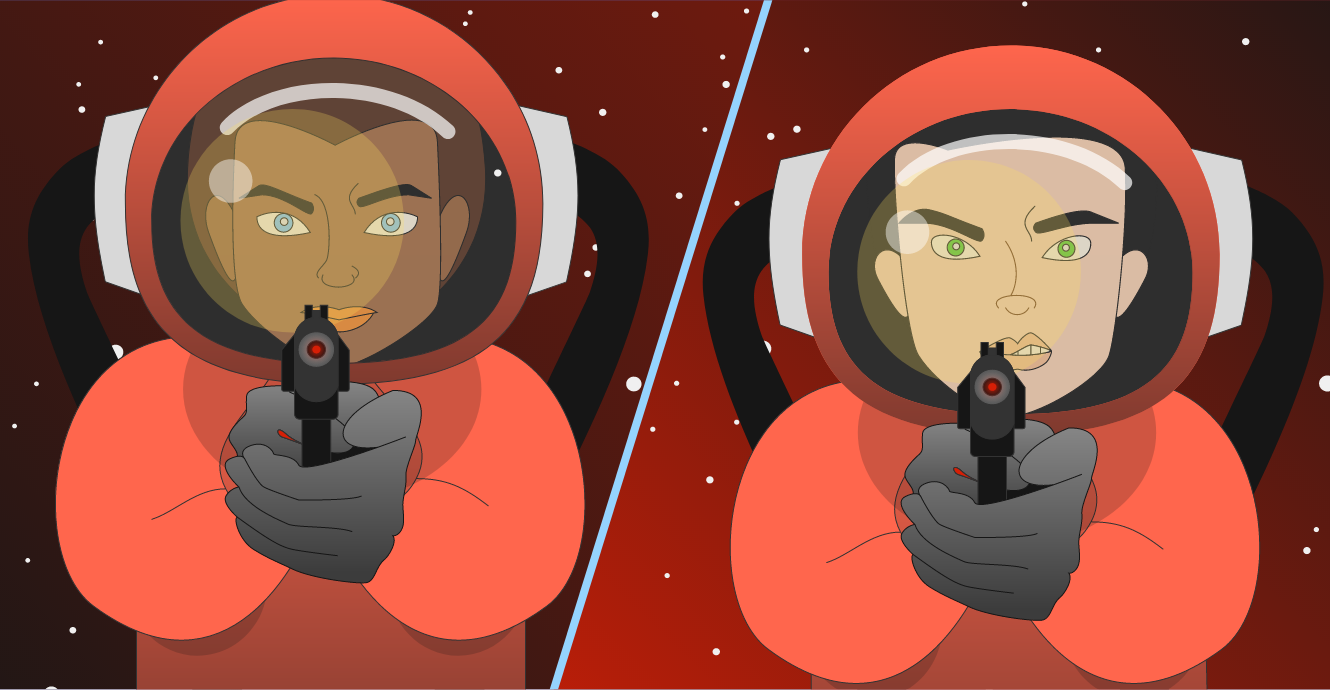Alyssa and Bev have set up a target halfway between them, and they are at rest relative to each other. The target is also at rest in their reference frame. Their goal is to coordinate their firing so that their lasers hit the target simultaneously.

Alyssa initiates the countdown to fire, and $T=0$ they both pull the trigger.

Do they see their laser bolts hitting the target at the same time?

Einstein's Postulates

Alyssa and Bev's friend Crater helps them move the targets around during practice, and he's always keeping an eye out to help them improve their game. He's usually on the move, and his jalopy shuttle runs at only one speed: out of control.

Crater observes the practice from a different reference frame, so he will possibly have a different take on events. So long as he doesn't turn or otherwise accelerate, his frame of reference is an inertial frame, and his observations are governed by Einstein's postulates.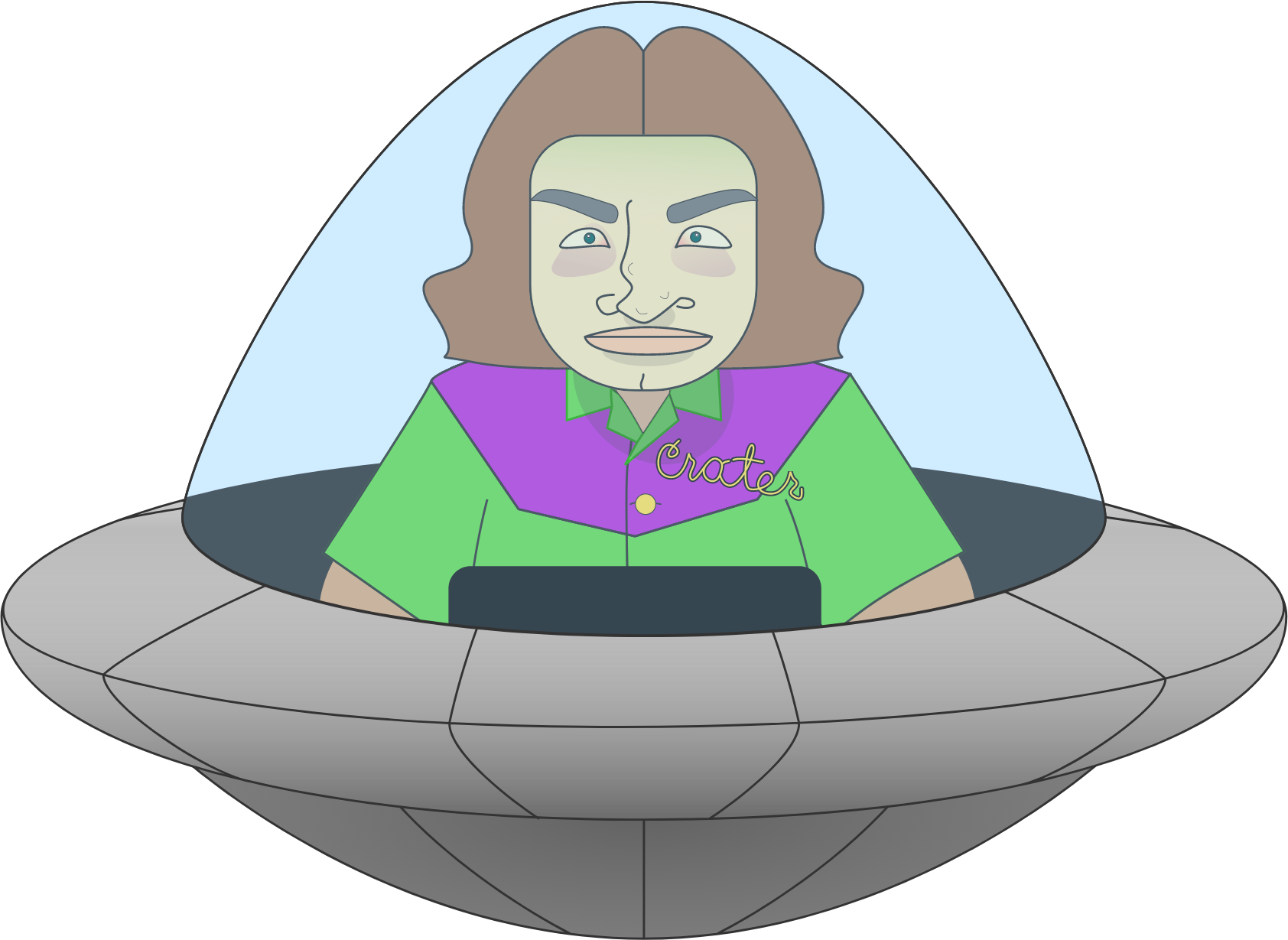Suppose Crater is heading directly toward Alyssa with speed $V$ (relative to her) at the instant she fires her laser. Appealing to Einstein's postulates, with what speed does the laser bolt approach Crater in his reference frame?

Einstein's Postulates

As Crater passes Alyssa with speed $V,$ he draws a spacetime diagram as they practice synchronizing their shots. In Crater's inertial frame, it's actually Alyssa and Bev who are moving with speed $V,$ and he draws their worldlines in blue.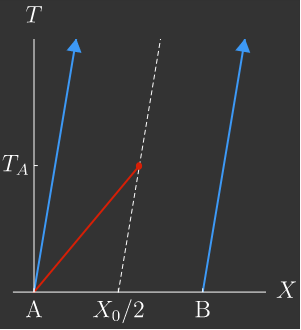Crater calls the time Alyssa fires $T=0$ in his frame of reference. At this moment, Crater measures the target at a distance of $X_0/2$ from his and Alyssa's position, $X=0.$

Crater adds Alyssa's shot to the diagram in red. At what time in Crater's frame $T_A$ does Alyssa's bolt hit the target?

Einstein's Postulates

Bev now takes a shot. According to Crater, does Bev's laser bolt take more time, less time, or the same amount of time to reach the target compared to Alyssa's?

Note: When Bev fires her laser, Crater also measures her at a distance $\frac{X_0}2$ from the target.

Einstein's Postulates

Crater reports to Alyssa and Bev on the comm link: "You aren't firing in sync." (Although, mysteriously, he sees the bolts hit the target at the same time.)

In order for the bolts to hit the target simultaneously in Crater's reference frame, how long after Alyssa fires $\Delta T$ does Bev need to pull the trigger?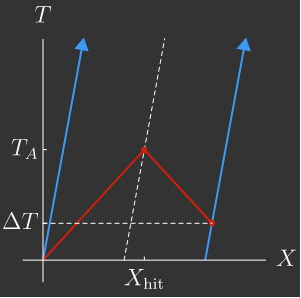Hint: A simultaneous hit means that the worldlines of Alyssa and Bev's laser bolts intersect the target's worldline at the same point $(X=X_\textrm{hit})$.

Einstein's Postulates

Alyssa gets on the comm link: "Crater, what are you talking about? We are firing at exactly the same time!"

Puzzled, Crater does an experiment. He hangs a U-turn in his shuttle and heads back toward Bev. Now, according to Crater, whose shot will require less time to hit the target?

Einstein's Postulates

If light propagates at the same speed for all inertial observers, as Einstein asserted, we arrive quickly at some pretty bizarre conclusions.

According to Crater, who is moving relative to the target, Bev and Alyssa fire at different times, yet their bolts are simultaneous on the target when the target is equidistant from them.

Perhaps even stranger, the person who fires first switches if Crater changes directions.

Imagine it's 1905, during the height of aether hysteria, and your colleague Einstein and you have the following conversation:

"Listen, I've got a theory of light propagation that doesn't require aether."

Marvelous!

"In my theory, observers experience events in a different order depending on which direction they're moving."

...Rats!

We just finished our first calculation of Einstein's relativistic theory. However bizarre it may seem, Einstein's theory of special relativity has been tested repeatedly, and all of its phenomena have been definitively confirmed.

Let's continue our journey, shall we?

Einstein's Postulates

Alyssa and Bev fire their lasers at the same time, but we were able to show using Einstein's postulates that in Crater's reference frame, these two events are not simultaneous.

At the laser tag competition, Crater will help Alyssa and Bev know where and when to fire, so she wants to map the coordinates of Alyssa's and Bev's reference frame $($which she calls $X^\prime$ and $T^\prime)$ into Crater's $X$ and $T$ coordinate system.

Alyssa would like to start with figuring out where her coordinate axes are on Crater's diagram. She designates her position as $X^\prime=0$ in her frame's coordinates. Crater's frame is moving with speed $V$ (from Bev toward Alyssa), so she sets her clock so that $T^\prime=0$ as Crater passes her position.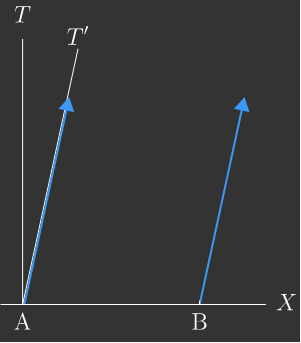What is the slope of Alyssa's $T^\prime$-axis on Crater's spacetime diagram?

Einstein's Postulates

Now Alyssa is working out how her $X^\prime$-axis runs through Crater's coordinate system.

Alyssa and Bev fire at the same time, $T^\prime=0,$ in their frame from different points on the $X^\prime$-axis. Therefore, on Crater's spacetime diagram, the $X^\prime$-axis must pass through the event corresponding to Bev firing her laser.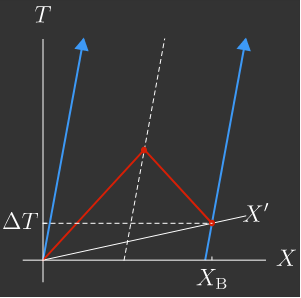Using your previous result for $\Delta T,$ work out Bev's $X$-coordinate $X_B$ in Crater's frame when she fires.

Recall: Bev is at $X=X_0$ in Crater's coordinate system when Alyssa fires her laser.

Einstein's Postulates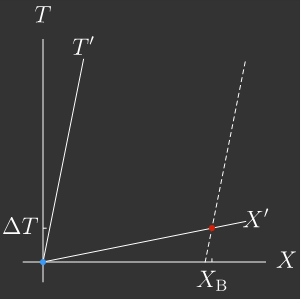The slope of the $X^\prime$-axis is the inverse of the $T^\prime$-axis slope: $\frac{\Delta T}{X_\text{B}}=\frac{V}{c^2}.$ The greater the relative speed between frame, the more the primed frame's coordinates are skewed.

Now Crater, Bev, and Alyssa can represent two different reference frames on the same spacetime diagram. By exploring the consequences of Einstein's second postulate that all inertial observers measure the same $c,$ they are well on their way to being able to relay the coordinates of events when they are moving relative to each other.

In the next quiz, we will complete their training and discover a formal transformation connecting coordinates in Crater's frame to the Alyssa and Bev's, but we already have an idea of what that will look like: the coordinates in a different reference frame are a mixture of spatial coordinate $X$ and time coordinate $T.$

Einstein's Postulates

×